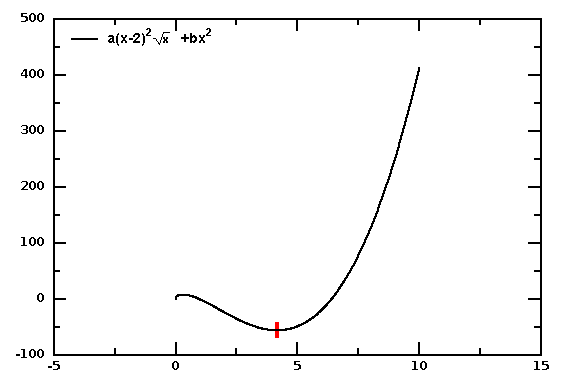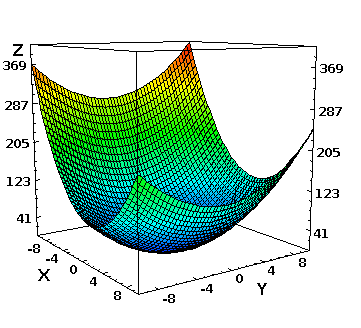DMelt:Numeric/6 Minimization

(diff) ← Older revision | Latest revision (diff) | Newer revision → (diff)
Limitted access. First login to DataMelt if you are a full DataMelt member. Then login to HandWiki as a user.

Minimization

Minimization is a subtopic is more broader "mathematical optimization" topic: Mathematical_optimization.

Minuit minimization

Any function that extendsjhplot.FNon can be minimized. How to define arbitrary complicated function in multiple dimensions was described before.

1D case

Let us give an example of how to find a minimum of a function shown in this figure:which is defined as $\displaystyle{ a(x-2)^2 * \sqrt{x}+b*x^2 }$. To minimize this function we will useJMinuitOptimizer. Here is a small script that finds and print the value of the variable $x$ which minimizes the function using the Migrad method:

Configuring the minimization

One can define the minimization methods, strategies, precision, tolerance and a maximum number of iterations. This is especially needed if the minimization fails. The above example uses the so-called Migrad method that requires the knowledge of first derivatives. Thus, it can fail if such knowledge is insufficient.

2D case

Minimization can be done for a function in any dimension, with any number of parameters. Let us consider 2D case, $\displaystyle{ a*(x-2)^2 + (y+1)^2 }$. The example script that performs minimization and plotting in 2D is shown below:

The output is (2,-1) and the generated image file is:This is an alternative approach. First we will consider a minimization of a function in 1D using theMnMigrad class.

1D case

Let us consider a trivial example of minimization for a function $\displaystyle{ 10+x^2 }$.

from org.freehep.math.minuit import *

class func(FCNBase): # define user functions
def valueOf(self, par):
return 10+par*par  # 10+x^2 function

Par = MnUserParameters()
state=vmin.userState()
print "Min value=:",vmin.fval(), "function calls=",vmin.nfcn()
print "Parameters=",state.params()

The output of this code is: <hidden click here to view the output>

Min value=: 10.0 function calls= 13
Parameters= array('d', [-2.1424395590941003e-10])
Minuit did successfully converge.
# of function calls: 13
minimum function value: 10.0000
minimum edm: 4.57506e-20
minimum internal state vector: LAVector parameters: -2.14244e-10
minimum internal covariance matrix: LASymMatrix parameters: 1.00000
# ext. ||   name    ||   type  ||   value   ||  error +/-
0 ||         x ||   free   || -2.14244e-10 ||    1.00000
MnUserCovariance:   1.00000
MnUserCovariance parameter correlations:  1.00000
MnGlobalCorrelationCoeff:  0.00000

Note that for a complicated function minimisation may fail. It is important to check that the minimisation is successful; if not, one can try alternative strategy of the Minuit. Modify the above code by including isValid() method:

......
if vmin.isValid()==False:  # try with higher strategy
.....

The above example can be run using Java codding as shown below:

import org.freehep.math.minuit.*;
public class Main {
public static void main(String[] args) {
FCNBase myFunction = new FCNBase() {
public double valueOf(double[] par) {
return 10 + par*par; } };

MnUserParameters myParameters = new MnUserParameters();
System.out.printf("Minimum value is %g found using %d function calls",
min.fval(),min.nfcn());
}  }

The above code needs to be modified in order to produce a graphic output. Let us consider a more complex example in which we will minimize the function $\displaystyle{ 10+x^2+\exp(x) }$. In order to plot such function and minimize at the same time, we will add additional method "addPlot()" to the function definition. This method returns the (X,Y) array for function plotting. This example shows how to minimize and plot this function. We also indicate the results of minimisation as a red dot:

2D case

Now let us consider a more complicated case of minimizing a function in 2D, which will also give you an idea how to do this for any arbitrary function in any dimension. We will consider a function $\displaystyle{ 100*(y-x^2)^2+(1-x)^2 }$. This function has the known minima at (1,1) with the Z value close to 0.

Constrained optimization

In this section we will discuss constrained optimization by linear approximation for any arbitrary function with any number of variables. The function can be constrained by certain condition. This algorithm is publicly available in the Jcobyla project but it was optimized in order to use it with scripting languages.

Let us consider a minimisation of a function with two variables. The function can be defined in Java as:

10.0 * Math.pow(x + 1.0, 2.0) + Math.pow(x, 2.0)

Let us minimize this function and determine a point (x,y) where the value of this function is smallest. We minimizes the objective function above with respect to a set of inequality constraints "CON". The function and CON may be non-linear, and should preferably be smooth.

The output of the above script is:

[-1.0, 0.0]

Note that the corresponding code in Java look as:

public void test01FindMinimum() {  // Java example
double rhobeg = 0.5;
double rhoend = 1.0e-6;
int iprint = 1;
int maxfun = 3500;

System.out.format("%nOutput from test problem 1 (Simple quadratic)%n");
Calcfc calcfc = new Calcfc() {
@Override
public double Compute(int n, int m, double[] x, double[] con) {
return 10.0 * Math.pow(x + 1.0, 2.0) + Math.pow(x, 2.0);
}
};
double[] x = {1.0, 1.0 };
CobylaExitStatus result = Cobyla.FindMinimum(calcfc, 2, 0, x, rhobeg, rhoend, iprint, maxfun);
}

The code illustrated that we overwrite the function Calcfc() method by using a custom code.

Let us make a more complicated example: a minimization of function with two variables in unit circle. This adds a constrain on the minimization. The example is shown below:

The next example shows minimization in three variables. We will minimize an ellipsoid (with one contain)